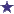# Alligation Question: 1.5L of 20% KCL to How Much of a 15% KCL Solution?Alligation Question on Potassium Chloride Solution...

A physician wants 1.5L of kcl 15% from a stock solution that is 20% kcl. How many ml of stock solution is needed?

### Comments for Alligation Question: 1.5L of 20% KCL to How Much of a 15% KCL Solution?

Average RatingRatingThis isn't one of my problems but I'll help anyway by: Anonymous When making tic tac toe table, 15% and 20%are what you have on hand and 1500 ml is your desired (1500 ml=1.5 L) You subtract 1500-.15=1499.85 and 1500-.20=1499.8=1499.Add those together (1499.8 +1499.85=2999.65) When you make your ratio/proportion table for first one 2999.65 x 1500/.20=2250 (estimated from 22497275) and second one 2999.65 x 1500/.15=3000 (estimated from 29996500) divide both by 1000 to get in L so 2250/1000=2.25 L and 3000/1000 is 3L of course ( or those could be 2.25 mL and 3 mL) Check textbook or ask instructor)